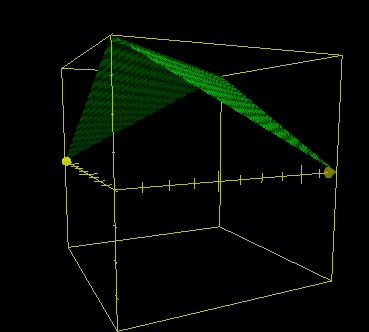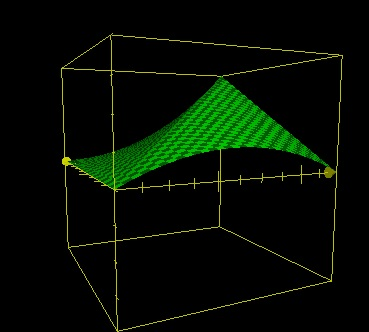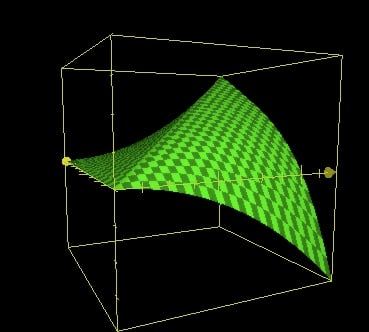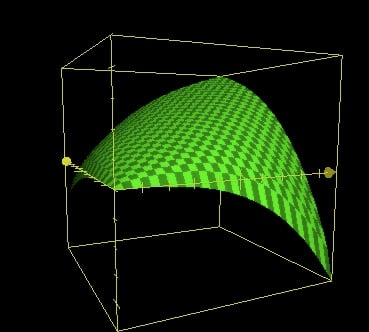# Working on the algorithm for Jamendo’s “similar albums”

I'm currently working on a big rewrite of the algorithms behind Jamendo's "Similar albums". The new algorithm will focus only on tags because there will be both "Similar albums" and "Related albums" (where I'll put recommended stuff). I was really slow this morning while thinking about it so I decided to blog the whole process in real time ;-)

Let's begin! The problem is, we have a list of weighted tags for each album and we want to compute for each album the list of album that have the most similar tags list.

Here is a sample tag/weight list for the Manu Cornet album:

```jazz            19.8494
latino          18.4662
bossa           16.4924
contrebasse     16.4924
sensible        16.4924
world           16
basse           15.6844
hamonica        15.6844
batterie        15.6844
tamtam          15.6844
improvisation   15.6844
piano           8.48528
easylistening   7.34847
ambient         6.63325
```

Let's call this base album "A1" and the similar albums we're looking for "A2". We can consider that "20" is the maximum tag weight.

A quick list of requirements for the algorithm :

• Shouldn't be symmetric
• Should penalize A2s if they lack one or more of the most important tags of A1
• Should be quite fast, even if we're not going to run it on the fly (everything will be computed once a day)

Martin told me yesterday about the nice app "Grapher" which is bundled with OS X. I'm going to use it to write the formula.

My first idea was, for each tag of A1, to compute a number Z between -1 and 1 and add them all. Let (x,y) be the weight of the tag in A1 and A2 respectively. The most obvious algorithm would be :z=20-ABS(x-y)

The axis on the left is Y, on the right it's X and the elevation is of course Z. The window is (0,20),(0,20),(-20,20)

Easy improvement : multiply by x/20z=(20-ABS(x-y))*x/20

Quite good but there is a problem over the X axis. If y=0, z should always be < 0. Another easy solution : add min(0,y-x)z=(20-ABS(x-y))*x/20+min(0,y-x)

New problem : x=20, y=10. In these conditions z shouldn't be nul. Let's multiply min(0,y-x) by (1-y/20).z=(20-ABS(x-y))*x/20+min(0,y-x)*(1-y/20)

Better, but not good enough yet. (1-y/20)^4 ?**z=(20-ABS(x-y))\*x/20+min(0,y-x)\*(1-y/20)\^4**

Well, it looks quite good to me! Maybe we could tweak some curves a bit but let's go for this one for now.

Here are the results for the Manu Cornet album :

```id                      weight_sum
----------------------- ------------------
2336                    89.7347740595245
608                     84.9463957742962
2013                    79.2737167470594
2239                    78.7533019317343
1968                    78.3494367998522
3552                    78.2419321761695
2626                    77.4643574361218
868                     76.3107863971299

(query took 1.2 seconds)
```

I am not so happy with the results. Maybe there need to be another negative zone for tags that are in A2 but not in A1 after all.

Let's reduce the window size to (0,1),(0,1),(-1,1) and take those "20" factors out. Now much easier, here is what I've come up :z=SQRT(x*y)+MIN(0,(y-x)^3)+0.5*MIN(0,(x-y)^3))

Looks good too but let's compare the results :

```id     weight_sum
------ ------------------
608    4.49465968951514
2336   4.32499417058527
2013   3.74828022088347
3552   3.49127205825584
2626   3.44471851383391
2407   3.35824086666307
2239   3.3511421181151
1968   3.27891762132192
868    3.18629627915296
1013   3.18123969513814
1741   3.11171873935927

(query took 1.2 seconds)
```

Quite funny that both queries take the same time to process: the formula doesn't seem to be the bottleneck. Results are a bit better but I think there will still be some tweaking needed in the future. Maybe reduce the negative zones.

Anyway, now I'm going to implement this and the new data should be online in a few minutes. Don't forget to check out the "Similar albums" slider on your favourite albums pages!